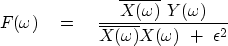Next: Explicit model for noise Up: HOW TO DIVIDE NOISY Previous: Example of deconvolution with

## Deconvolution with an unknown filter

Equation (12) solves Y=XF for X, giving the solution for what is called the deconvolution problem with a known wavelet F." We can also use Y=XF when the filter F is unknown, but the input X and output Y are given. Here stabilization might not be needed but would be necessary if the input and output did not fill the frequency band. Taking derivatives as above, but with respect to F instead of X, gives again equation (12) with X and F interchanged:(18)Next: Explicit model for noise Up: HOW TO DIVIDE NOISY Previous: Example of deconvolution with
Stanford Exploration Project
10/21/1998